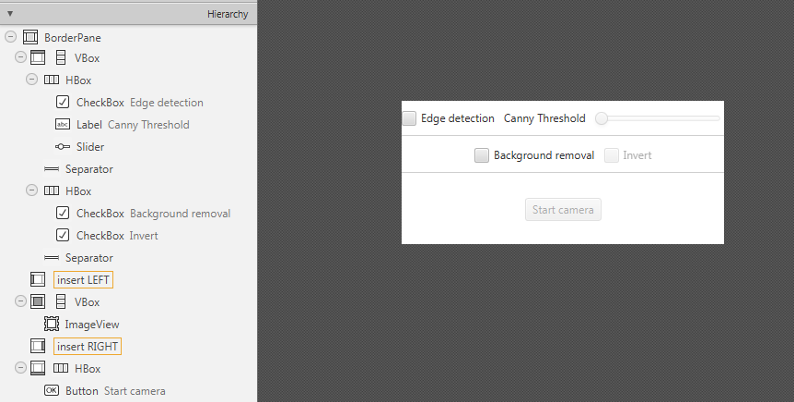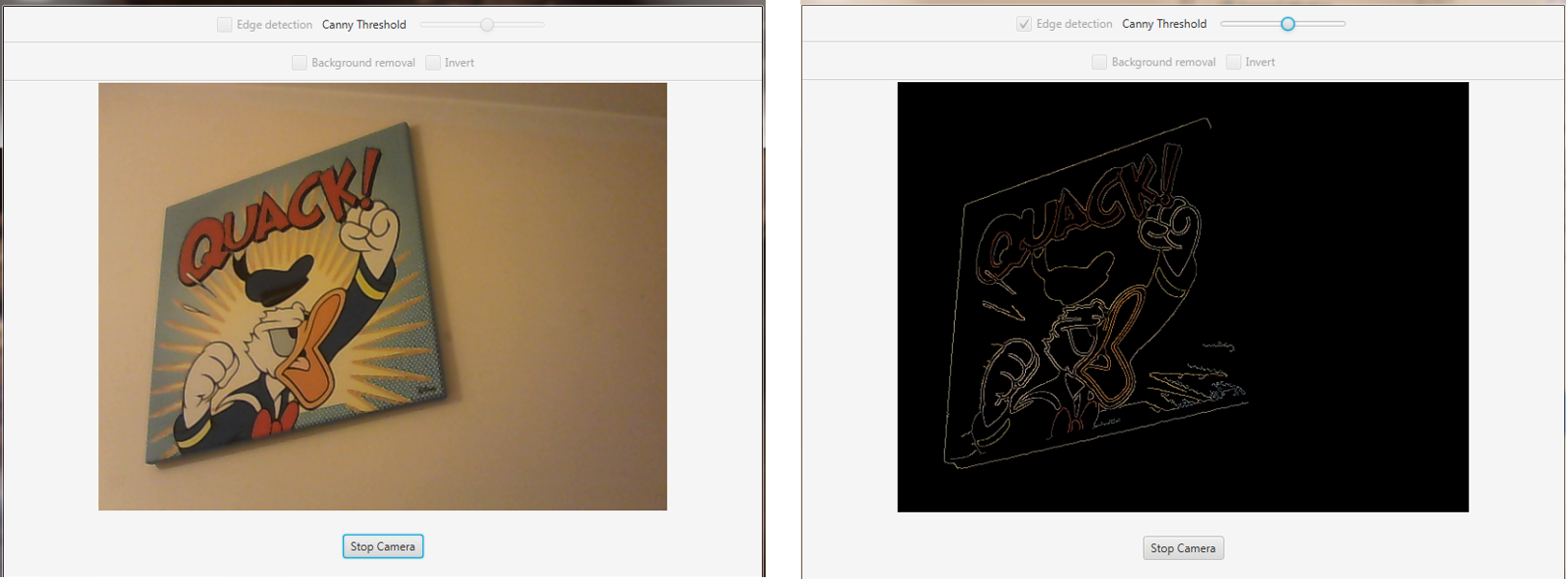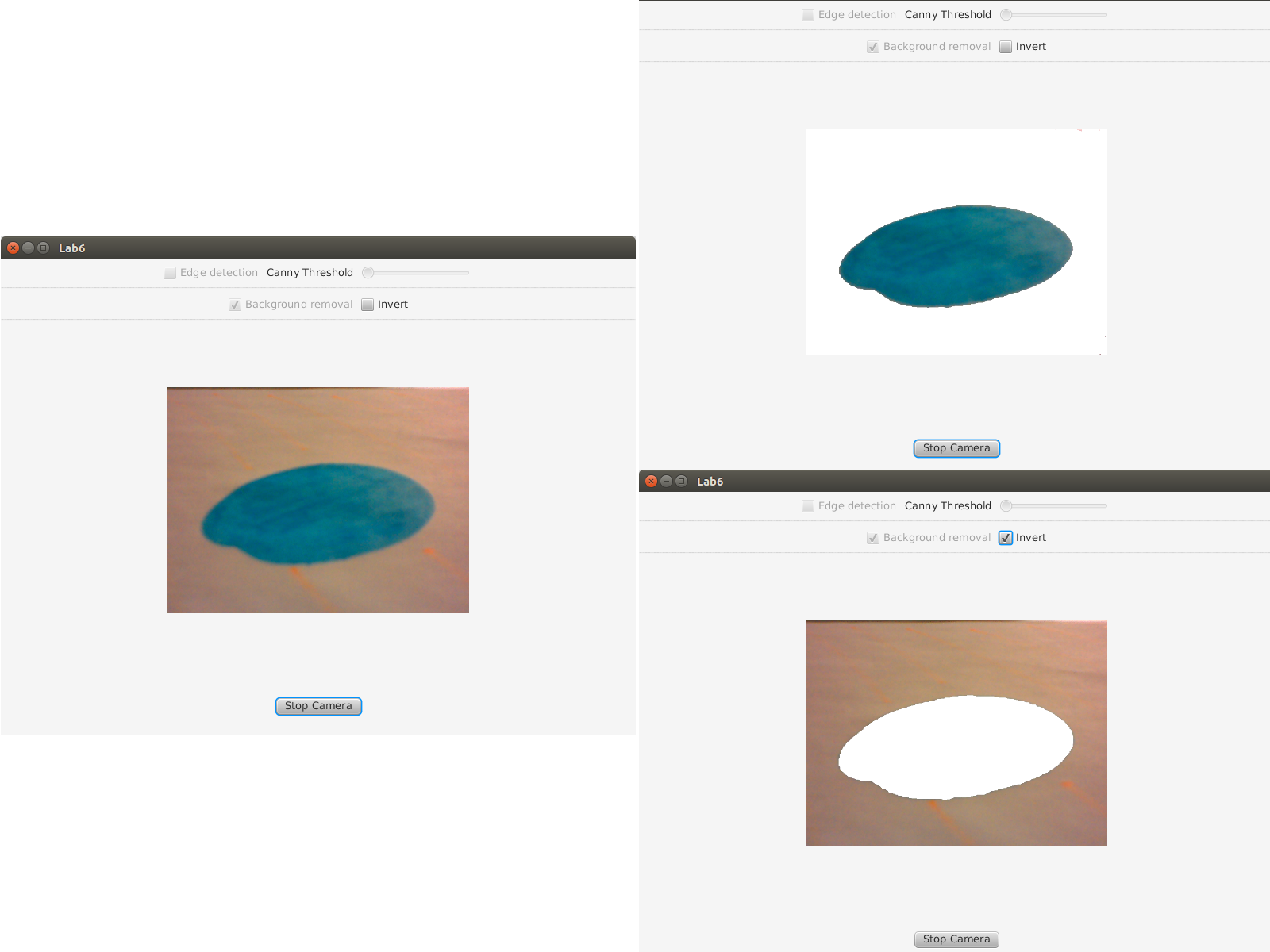# Image Segmentation¶

Note

We assume that by now you have already read the previous tutorials. If not, please check previous tutorials at http://opencv-java-tutorials.readthedocs.org/en/latest/index.html. You can also find the source code and resources at https://github.com/opencv-java/

## Goal¶

In this tutorial we are going to create a JavaFX application where we can decide to apply to video stream captured from our web cam either a Canny edge detector or a trivial background removal using the two basic morphological operations: dilatation and erosion.

## Canny edge detector¶

The Canny edge detector is an edge detection operator that uses a multi-stage algorithm to detect a wide range of edges in images. It was developed by John F. Canny in 1986.

Canny edge detection is a four step process:
1. A Gaussian blur is applied to clear any speckles and free the image of noise.
2. A gradient operator is applied for obtaining the gradients’ intensity and direction.
3. Non-maximum suppression determines if the pixel is a better candidate for an edge than its neighbors.
4. Hysteresis thresholding finds where edges begin and end.
The Canny algorithm contains a number of adjustable parameters, which can affect the computation time and effectiveness of the algorithm.
• The size of the Gaussian filter: the smoothing filter used in the first stage directly affects the results of the Canny algorithm. Smaller filters cause less blurring, and allow detection of small, sharp lines. A larger filter causes more blurring, smearing out the value of a given pixel over a larger area of the image.
• Thresholds: A threshold set too high can miss important information. On the other hand, a threshold set too low will falsely identify irrelevant information (such as noise) as important. It is difficult to give a generic threshold that works well on all images. No tried and tested approach to this problem yet exists.

For our purpose we are going to set the filter size to 3 and the threshold editable by a Slider.

## Dilatation and Erosion¶

Dilation and erosion are the most basic morphological operations. Dilation adds pixels to the boundaries of objects in an image, while erosion removes pixels on object boundaries. The number of pixels added or removed from the objects in an image depends on the size and shape of the structuring element used to process the image. In the morphological dilation and erosion operations, the state of any given pixel in the output image is determined by applying a rule to the corresponding pixel and its neighbors in the input image. The rule used to process the pixels defines the operation as a dilation or an erosion.

Dilatation: the value of the output pixel is the maximum value of all the pixels in the input pixel’s neighborhood. In a binary image, if any of the pixels is set to the value 1, the output pixel is set to 1.

Erosion: the value of the output pixel is the minimum value of all the pixels in the input pixel’s neighborhood. In a binary image, if any of the pixels is set to 0, the output pixel is set to 0.

## What we will do in this tutorial¶

In this guide, we will:
• Add a checkbox and a Slider to select and control the Canny edge detector.
• Use the Canny edge function provided by OpenCV.
• Use the Dilatation and erosion functions provided by OpenCV.
• Create a trivial background removal function.

## Getting Started¶

Let’s create a new JavaFX project. In Scene Builder set the windows element so that we have a Border Pane with:

• on TOP a VBox containing two HBox, each one followed by a separator.
• In the first HBox we are goning to need a checkbox and a slider, the first one is to select the Canny e.d. mode and the second one is going to be used to control the value of the threshold to be passed to the Canny e.d. function.

<CheckBox fx:id="canny" onAction="#cannySelected" text="Edge detection"/>
<Label text="Canny Threshold" />
<Slider fx:id="threshold" disable="true" />

• In the second HBox we need two checkboxes, the first one to select the Backgrond removal mode and the second one to say if we want to invert the algorithm (a sort of “foreground removal”).

<CheckBox fx:id="dilateErode" onAction="#dilateErodeSelected" text="Background removal"/>
<CheckBox fx:id="inverse" text="Invert" disable="true"/>

• in the CENTRE we are going to put an ImageView for the web cam stream.
<ImageView fx:id="originalFrame" />

• on the BOTTOM we can add the usual button to start/stop the stream
<Button fx:id="cameraButton" alignment="center" text="Start camera" onAction="#startCamera" disable="true" />


The gui will look something like this one:## Using the Canny edge detection¶

If we selected the Canny checkbox we can perform the method doCanny.

if (this.canny.isSelected()){
frame = this.doCanny(frame);
}


doCanny is a method that we define to execute the edge detection. First, we convert the image into a grayscale one and blur it with a filter of kernel size 3:

Imgproc.cvtColor(frame, grayImage, Imgproc.COLOR_BGR2GRAY);
Imgproc.blur(grayImage, detectedEdges, new Size(3, 3));


Second, we apply the OpenCV function Canny:

Imgproc.Canny(detectedEdges, detectedEdges, this.threshold.getValue(), this.threshold.getValue() * 3, 3, false);

where the arguments are:
• detectedEdges: Source image, grayscale
• detectedEdges: Output of the detector (can be the same as the input)
• this.threshold.getValue(): The value entered by the user moving the Slider
• this.threshold.getValue() * 3: Set in the program as three times the lower threshold (following Canny’s recommendation)
• 3: The size of the Sobel kernel to be used internally
• false: a flag, indicating whether to use a more accurate calculation of the magnitude gradient.

Then we fill a dest image with zeros (meaning the image is completely black).

Mat dest = new Mat();


Finally, we will use the function copyTo to map only the areas of the image that are identified as edges (on a black background).

frame.copyTo(dest, detectedEdges);


copyTo copies the src image onto dest. However, it will only copy the pixels in the locations where they have non-zero values.

## Canny Result¶## Using the Background Removal¶

If we selected the background removal checkbox we can perform the method doBackgroundRemoval

else if (this.dilateErode.isSelected())
{
frame = this.doBackgroundRemoval(frame);
}


doBackgroundRemoval is a method that we define to execute the background removal.

First we need to convert the current frame in HSV:

hsvImg.create(frame.size(), CvType.CV_8U);
Imgproc.cvtColor(frame, hsvImg, Imgproc.COLOR_BGR2HSV);
Now let's split the three channels of the image:
Core.split(hsvImg, hsvPlanes);


Calculate the Hue component mean value:

Imgproc.calcHist(hue, new MatOfInt(0), new Mat(), hist_hue, histSize, new MatOfFloat(0, 179));
for (int h = 0; h < 180; h++)
average += (hist_hue.get(h, 0) * h);
average = average / hsvImg.size().height / hsvImg.size().width;


If the background is uniform and fills most of the frame, its value should be close to mean just calculated. Then we can use the mean as the threshold to separate the background from the foreground, depending on the invert checkbox we need to perform a back(fore)ground removal:

 if (this.inverse.isSelected())
Imgproc.threshold(hsvPlanes.get(0), thresholdImg, threshValue, 179.0, Imgproc.THRESH_BINARY_INV);
else
Imgproc.threshold(hsvPlanes.get(0), thresholdImg, threshValue, 179.0, Imgproc.THRESH_BINARY);


Now we apply a low pass filter (blur) with a 5x5 kernel mask to enhance the result:

Imgproc.blur(thresholdImg, thresholdImg, new Size(5, 5));


Finally apply the dilatation then the erosion (closing) to the image:

Imgproc.dilate(thresholdImg, thresholdImg, new Mat(), new Point(-1, -1), 1);
Imgproc.erode(thresholdImg, thresholdImg, new Mat(), new Point(-1, -1), 3);

The functions take these parameters:
• thresholdImg input image;
• thresholdImg output image of the same size and type as thresholdImg;
• new Mat() a kernel;
• new Point(-1, -1) position of the anchor within the element; default value ( -1, -1 ) means that the anchor is at the element center.
• 6 number of times the operation is applied.

After the closing we need to do a new binary threshold:

Imgproc.threshold(thresholdImg, thresholdImg, threshValue, 179.0, Imgproc.THRESH_BINARY);


At last, we can apply the image we’ve just obtained as a mask to the original frame:

Mat foreground = new Mat(frame.size(), CvType.CV_8UC3, new Scalar(255, 255, 255));
frame.copyTo(foreground, thresholdImg);


## Background Removal Result¶The source code of the entire tutorial is available on GitHub.